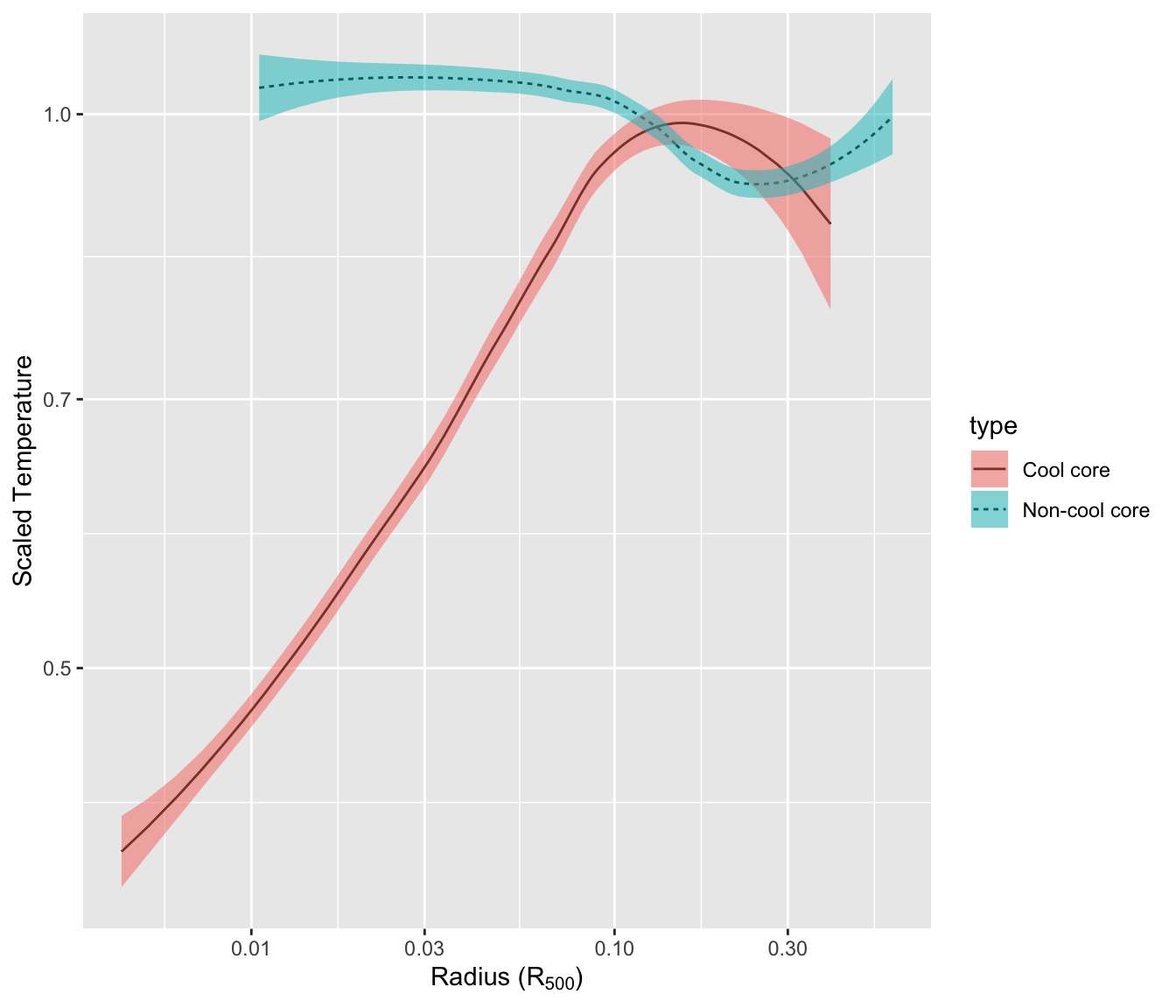# Line chart with error envelop: ggplot2 and geom_ribbon()

This post explains how to add an error envelop around a line chart using `ggplot2` and the `geom_ribbon()` function.

# Basic line chart with `ggplot2` and `geom_line()`

This graph has been made by Alastair Sanderson. You can have a look to his gallery here.

It shows mean temperature profiles and their error envelopes, using the ggplot2 package and its `geom_ribbon()` function.

Note that `geom_ribbon` is used since upper and lower values of the envelop are available in the input data. As an alternative, the geom_smooth function autamatically draw an error envelop using different statistical models.``````library(ggplot2)

# Get the data from the web !
CC\$type <- "Cool core"
nCC\$type <- "Non-cool core"
A <- rbind(CC, nCC)

# Make the plot
ggplot(data=A, aes(x=r.r500, y=sckT, ymin=sckT.lo, ymax=sckT.up, fill=type, linetype=type)) +
geom_line() +
geom_ribbon(alpha=0.5) +
scale_x_log10() +
scale_y_log10() +
xlab(as.expression(expression( paste("Radius (", R, ")") ))) +
ylab("Scaled Temperature")``````

Related chart types

## Contact

This document is a work by Yan Holtz. Any feedback is highly encouraged. You can fill an issue on Github, drop me a message on Twitter, or send an email pasting yan.holtz.data with gmail.com.